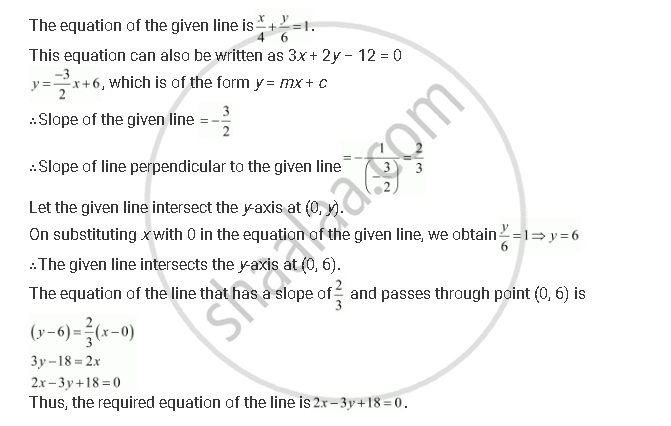CBSE (Arts) Class 11CBSE
Share

# Find the Equation of a Line Drawn Perpendicular to the Line X/4 + Y/6 = 1Through the Point, Where It Meets The Y-axis. - CBSE (Arts) Class 11 - Mathematics

#### Question

Find the equation of a line drawn perpendicular to the line x/4 + y/6 = 1through the point, where it meets the y-axis.

#### SolutionIs there an error in this question or solution?

#### Video TutorialsVIEW ALL 

Solution Find the Equation of a Line Drawn Perpendicular to the Line X/4 + Y/6 = 1Through the Point, Where It Meets The Y-axis. Concept: Slope of a Line.
S